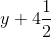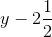# Algebra (Exercise 11.4)## EX 11.4 QUESTION 1.

(a) Take Sarita’s present age to be y years

(i) What will be her age 5 years from now?

(ii) What was her age 3 years back?

(iii) Sarita’s grandfather is 6 times her age. What is the age of her grandfather?

(iv) Grandmother is two year younger than grandfather. What is grandmother’s age?

(v) Sarita’s father’s age is 5 years more than 3 times Sarita’s age. What is her father’s age?

(b) The length of a rectangular hall is 4 meters less than three times the breadth of the hall. What is the length, if the breadth is b meters?

(c) A rectangular box has height h cm. Its length is 5 times the height and breadth is 10 cm less than the length. Express the length and the breadth of the box in terms of the height.

(d) Meena, Beena and Reena are climbing the steps to the hill top. Meena is at step s, Beena is 8 steps ahead and Leena 7 steps behind. Where are Beena and Meena? The total number of steps to the hill top is 10 less than 4 times what Meena has reached. Express the total number of steps using s.

(e) A bus travels at v km per hour. It is going from Daspur to Beespur. After the bus has travelled 5 hours, Beespur is still 20 km away. What is the distance from Daspur to Beespur? Express it using v.

`Solution:

(a) (i) y + 5

(ii) y – 3

(iii) 6y

(iv) 6y – 2

(v) 3y + 5

(b)  length = 3b

breadth = (3b – 4) metre

(c) Height of the rectangular box = h

Length = 5h cm

Breadth = 10 cm less  than length

=(5h – 10) cm.

(d) Meena’s position = s

Beena’s position 8 step ahead =  s + 8

Leena’s position 7 step behind = s – 7

Total number of steps = 4s – 10

(d) Speed of bus = v km/h

Distance travelled by Bus in 5 hours = 5v km.

Remaining distance = 20 km

∴ Distance from Daspur to Beespur = (5v + 20) km.

## EX 11.4 QUESTION 2.

Change the following statements using expressions into statements in ordinary language.

(For example, Given Salim scores r runs in a cricket match, Nalin scores (r + 15) runs. In ordinary language – Nalin scores 15 runs more than Salim.)

(a) A notebook costs ₹ p. A book costs ₹ 3p

(b) Tony put q marbles on the table. He has 8 q marbles in his box.

(c) Our class has n students. The school has 20 n students.

(d) Jaggu is z years old. His uncle is 4z years old and his aunt is (4z – 3) years old.

(e) In an arrangement of dots there are r rows. Each row contains 5 dots

Solution:

(a) A book costs 3 times the cost of a notebook.

(b) The number of marbles in the box is 8 times the marbles on the table.

(c) The school has 20 times the number of students in a class.

(d) Jaggu’s uncle’s age is 4 times the age of jaggu. and his aunt’s age is 3 years less than the age of his uncle.

(e) The total number of dots is 5 times the number of rows.

## EX 11.4 QUESTION 3.

(a) Given Munnu’s age to be x years, can you guess what (x – 2) may show?

Can you guess what (x + 4) may show? What (3x + 7) may show?

(b) Given Sara’s age today to be y years. Think of her age in the future or in the past.

What will the following expression indicate? Y + 7, y – 3,(c) Given n students in the class like football, what may 2n shows? What may n / 2 show?

Solution:

(a) Munnu’s age = x years

∴ (x -2) years may be the age of her younger brother or younger sister.
(x + 4) years show the age of her elder brother or elder sister.
(3x + 7) years may be the age of her father, mother, or uncle.

(b) Sara’s today age= y years.

y + 7 shows her future age.

y – 3 shows her past age.

y + 4$\frac { 1 }{ 2 }$  show her future age i.e., the age after z

four and a half years.

y – 2$\frac { 1 }{ 2 }$ shows her past age i.e., the age before two and a half years.

(c) Number of students who like football = n

∴ 2n = twice the number of football players may like to play cricket.

and$\frac { n }{ 2 }$ = half of the number of football 2

players may like to play basketball.

error: Content is protected !!